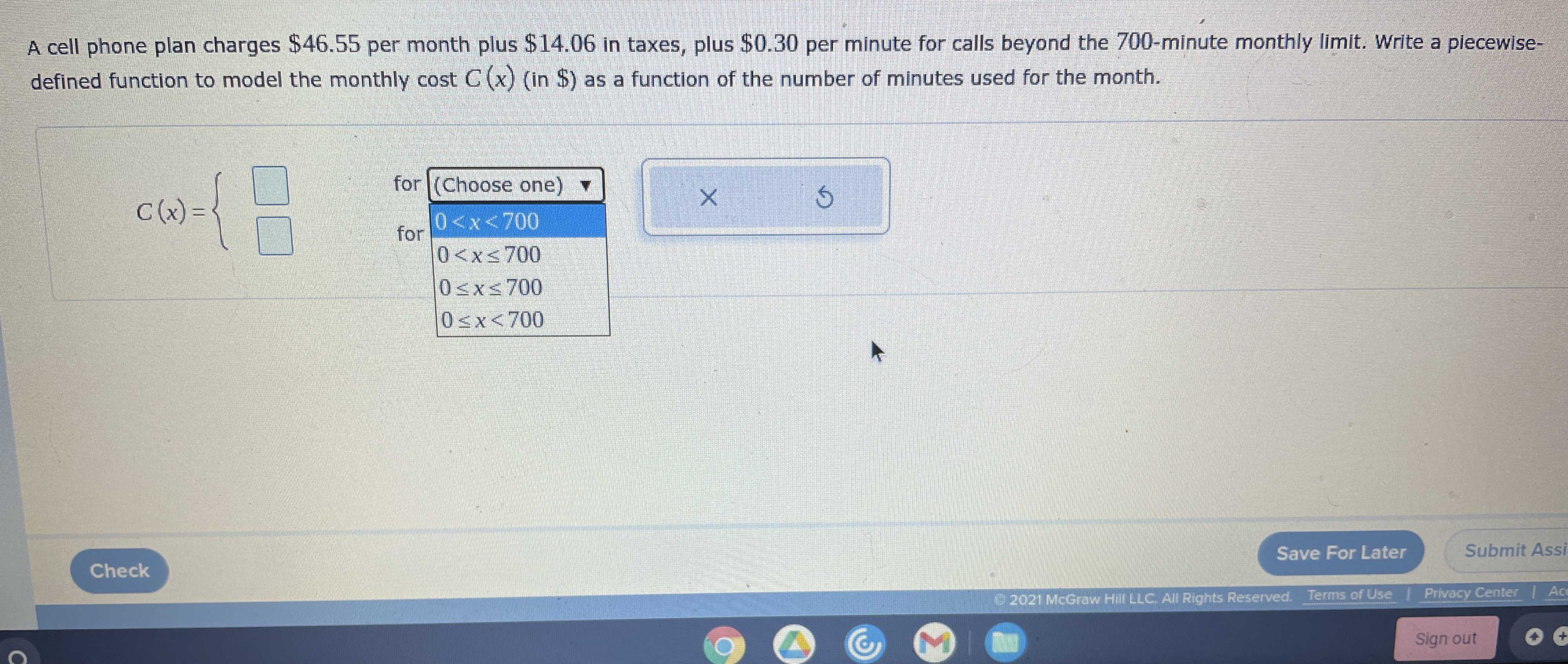### ¿Todavía tienes preguntas de matemáticas?

Pregunte a nuestros tutores expertos
Algebra
PreguntaA cell phone plan charges $$\ 46.55$$ per month plus $$\ 14.06$$ in taxes, plus $$\ 0.30$$ per minute for calls beyond the $$700$$ -minute monthly limit. Write a piecewise- defined function to model the monthly cost $$C ( x )$$ (in $$\$$ ) as a function of the number of minutes used for the month.

$$C(x)= 60.61,0\le x\le 700\\C(x)= =0.3x- 149.39,x> 700$$